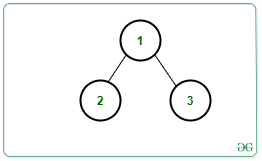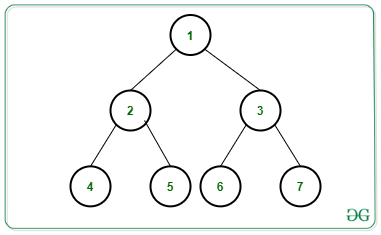# Count root to leaf paths having exactly K distinct nodes in a Binary Tree

Given a Binary Tree consisting of N nodes rooted at 1, an integer K and an array arr[] consisting of values assigned to each node, the task is to count the number of root to leaf paths having exactly K distinct nodes in the given Binary Tree.

Examples:

Input: N = 3, Edges[][] = {{1, 2}, {1, 3}}, arr[] = {3, 3, 2}, K = 2, Below is the given Tree:Output: 1
Explanation:
There exists only 1 distinct path i.e., Path 1 -> 3 contains 2 distinct nodes.

Input: N = 7, Edges[][] = {{1, 2}, {1, 3}, {2, 4}, {2, 5}, {3, 6}, {3, 7}}, arr[] = {2, 2, 2, 2, 3, 5, 2}, K = 1, Below is the given Tree:Output: 2
Explanation:
There exists only 2 distinct paths containing 1 distinct node:
1) Paths 1 -> 2 -> 4,
2) Paths 1 -> 3 -> 7

Naive Approach: The simplest approach is to generate all possible paths from the root to the leaf nodes and for each path, check if it contains K distinct nodes or not. Finally, print the count of such paths.
Time Complexity: O(N * H2), where H denotes the height of the tree.
Auxiliary Space: O(N);

Efficient Approach: The idea is to use Preorder Traversal and a Map to count the distinct node in the path from the root to the current node. Follow the below steps to solve the problem:

• Initialize a variable distinct_nodes as 0 to store the count of the distinct node from the root to the current node and ans as 0 to store the total distinct root to leaf paths having K distinct node.
• Perform Preorder Traversal in the given binary tree and store the count of the distinct node from root to the current node in the map M.
• Whenever a node occurs for the first time on a path, increase the count of distinct nodes by 1.
• If the count of distinct nodes on a path becomes greater than K return to the parent node of the current node.
• Otherwise, continue to visit the children of the current node incrementing the frequency of the current node value by 1.
• In the above step, increment ans by 1 if the count of distinct nodes on that root to leaf path is exactly equal to K.
• After the above steps print the value of ans as the resultant count.

Below is the implementation of the above approach:

## C++

 `// C++ program for the above approach` `#include ` `using` `namespace` `std;`   `// Structure of a Tree Node` `struct` `Node {` `    ``int` `key;` `    ``Node *left, *right;` `};`   `// Function to create new tree node` `Node* newNode(``int` `key)` `{` `    ``Node* temp = ``new` `Node;` `    ``temp->key = key;` `    ``temp->left = temp->right = NULL;` `    ``return` `temp;` `}`   `// Function to count all root to leaf` `// paths having K distinct nodes` `void` `findkDistinctNodePaths(` `    ``Node* root, unordered_map<``int``, ``int``> freq,` `    ``int` `distinct_nodes, ``int` `k, ``int``& ans)` `{` `    ``// If current node is null` `    ``if` `(root == NULL)` `        ``return``;`   `    ``// Update count of distinct nodes` `    ``if` `(freq[root->key] == 0)` `        ``distinct_nodes++;`   `    ``// If count > k then return to` `    ``// the parent node` `    ``if` `(distinct_nodes > k)` `        ``return``;`   `    ``// Update frequency of current node` `    ``freq[root->key]++;`   `    ``// Go to the left subtree` `    ``findkDistinctNodePaths(root->left,` `                           ``freq,` `                           ``distinct_nodes,` `                           ``k, ans);`   `    ``// Go to the right subtree` `    ``findkDistinctNodePaths(root->right,` `                           ``freq,` `                           ``distinct_nodes,` `                           ``k, ans);`   `    ``// If current node is leaf node` `    ``if` `(root->left == NULL` `        ``&& root->right == NULL) {`   `        ``// If count of distinct node` `        ``// is same as K, increment ans` `        ``if` `(distinct_nodes == k)` `            ``ans++;` `    ``}` `}`   `// Function to find count of root to` `// leaf paths having K distinct node` `void` `printkDistinctNodePaths(Node* root,` `                             ``int` `k)` `{` `    ``// Initialize unordered map` `    ``unordered_map<``int``, ``int``> freq;`   `    ``// Stores count of distinct node` `    ``int` `distinct_nodes = 0;`   `    ``// Stores total count of nodes` `    ``int` `ans = 0;`   `    ``// Perform Preorder Traversal` `    ``findkDistinctNodePaths(root, freq,` `                           ``distinct_nodes,` `                           ``k, ans);`   `    ``// Print the final count` `    ``cout << ans;` `}`   `// Driver Code` `int` `main()` `{` `    ``/*         2` `             ``/   \` `            ``/     \` `           ``1       3` `          ``/ \     /  \` `         ``/   \   /    \` `        ``4     2 -5     3` `    ``*/`   `    ``// Given Binary Tree` `    ``Node* root = newNode(2);` `    ``root->left = newNode(1);` `    ``root->right = newNode(3);` `    ``root->left->left = newNode(4);` `    ``root->left->right = newNode(2);` `    ``root->right->left = newNode(-5);` `    ``root->right->right = newNode(3);`   `    ``// Given K` `    ``int` `K = 2;`   `    ``// Function Call` `    ``printkDistinctNodePaths(root, K);`   `    ``return` `0;` `}`

## Java

 `// Java program for the ` `// above approach` `import` `java.util.*;` `class` `GFG{`   `// Structure of a ` `// Tree Node` `static` `class` `Node ` `{` `  ``int` `key;` `  ``Node left, right;` `};` `  `  `static` `int` `ans;` `  `  `// Function to create ` `// new tree node` `static` `Node newNode(``int` `key)` `{` `  ``Node temp = ``new` `Node();` `  ``temp.key = key;` `  ``temp.left = temp.right = ``null``;` `  ``return` `temp;` `}`   `// Function to count all root ` `// to leaf paths having K ` `// distinct nodes` `static` `void` `findkDistinctNodePaths(Node root, ` `                                   ``HashMap freq, ` `                                   ``int` `distinct_nodes, ` `                                   ``int` `k)` `{` `  ``// If current node is null` `  ``if` `(root == ``null``)` `    ``return``;`   `  ``// Update count of distinct nodes` `  ``if` `(!freq.containsKey(root.key))` `    ``distinct_nodes++;`   `  ``// If count > k then return ` `  ``// to the parent node` `  ``if` `(distinct_nodes > k)` `    ``return``;`   `  ``// Update frequency of ` `  ``// current node` `  ``if``(freq.containsKey(root.key))` `  ``{` `    ``freq.put(root.key, ` `    ``freq.get(root.key) + ``1``);` `  ``}` `  ``else` `  ``{` `    ``freq.put(root.key, ``1``);` `  ``}`   `  ``// Go to the left subtree` `  ``findkDistinctNodePaths(root.left, freq,` `                         ``distinct_nodes, k);`   `  ``// Go to the right subtree` `  ``findkDistinctNodePaths(root.right, freq,` `                         ``distinct_nodes, k);`   `  ``// If current node is ` `  ``// leaf node` `  ``if` `(root.left == ``null` `&& ` `      ``root.right == ``null``) ` `  ``{` `    ``// If count of distinct node` `    ``// is same as K, increment ans` `    ``if` `(distinct_nodes == k)` `      ``ans++;` `  ``}` `}`   `// Function to find count of root to` `// leaf paths having K distinct node` `static` `void` `printkDistinctNodePaths(Node root, ` `                                    ``int` `k)` `{` `  ``// Initialize unordered map` `  ``HashMap freq = ``new` `HashMap<>();`   `  ``// Stores count of ` `  ``// distinct node` `  ``int` `distinct_nodes = ``0``;`   `  ``// Stores total ` `  ``// count of nodes` `  ``ans = ``0``;`   `  ``// Perform Preorder Traversal` `  ``findkDistinctNodePaths(root, freq,` `                         ``distinct_nodes, k);`   `  ``// Print the final count` `  ``System.out.print(ans);` `}`   `// Driver Code` `public` `static` `void` `main(String[] args)` `{` `  ``/*           2` `             ``/   \` `            ``/     \` `           ``1       3` `          ``/ \     /  \` `         ``/   \   /    \` `        ``4     2 -5     3` `    ``*/`   `  ``// Given Binary Tree` `  ``Node root = newNode(``2``);` `  ``root.left = newNode(``1``);` `  ``root.right = newNode(``3``);` `  ``root.left.left = newNode(``4``);` `  ``root.left.right = newNode(``2``);` `  ``root.right.left = newNode(-``5``);` `  ``root.right.right = newNode(``3``);`   `  ``// Given K` `  ``int` `K = ``2``;`   `  ``// Function Call` `  ``printkDistinctNodePaths(root, K);` `}` `}`   `// This code is contributed by gauravrajput1`

Output:

```2

```

Time Complexity: O(N)
Auxiliary Space: O(N)

Attention reader! Don’t stop learning now. Get hold of all the important DSA concepts with the DSA Self Paced Course at a student-friendly price and become industry ready.

My Personal Notes arrow_drop_upCheck out this Author's contributed articles.

If you like GeeksforGeeks and would like to contribute, you can also write an article using contribute.geeksforgeeks.org or mail your article to contribute@geeksforgeeks.org. See your article appearing on the GeeksforGeeks main page and help other Geeks.

Please Improve this article if you find anything incorrect by clicking on the "Improve Article" button below.

Improved By : GauravRajput1

Article Tags :
Practice Tags :

1

Please write to us at contribute@geeksforgeeks.org to report any issue with the above content.